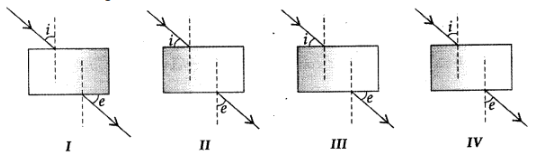# Mcq on refraction of light physics class 10 -with-answers

Question 1. The quantity which does not changes during refraction is

Explanation:-
Frequency of light does not change on refraction , When a ray of light passes from one medium to another, its direction (or path) changes because of change in speed of light from one medium to another.
Question 2. The refractive index of a medium is

Answer : A) Speed of light in air to speed of light in medium
Explanation:-
The refractive index is the measure of bending of a light ray when passing from one medium to another. It can also be defined as the ratio of the velocity of a light ray in an empty space to the velocity of light in a substance, n = c/v.
Question 3. The bending of light as it passes from one medium to another is called

Explanation:-
The bending of light as it passes from one medium to another is called refraction. It happens due to change in speed/velocity of light as it passes from one medium to another medium.

Question 4. The ratio of sin (x°) of the angle of incidence to sin (x°) of the angle of refraction is

Explanation:-
The ratio of sin (x°) of the angle of incidence to sin (x°) of the angle of refraction is called Snell's law.
Question 5. A ray of light is travelling from a rarer medium to a denser medium. While entering the denser medium at the point of incidence, it

Answer: B) bends towards the normal.
Explanation:-
Refraction is the bending of light rays after entering a medium where its speed is different. Due to refraction of light, when a ray of light passes from a rarer medium to a denser medium, bends towards the normal to the boundary between the two media.
Question 6. A student does the experiment on tracing the path of a ray of light passing through a rectangular glass slab for different angles of incidence. He can get a correct measure of the angle of incidence and the angle of emergence by following the labelling indicated in figure:Explanation:-
The angle formed by the incident ray and the normal to the rectangular slab is taken as the angle of incidence and the angle formed by the emergent ray and the normal is taken as the angle of emergence. Angle of incidence and angle of emergence will be same as both side of glass slab, the medium is air.
Question 7. A ray of light is travelling in a direction perpendicular to the boundary of a parallel glass slab. The ray of light:

Answer: D) Does not get refracted
Explanation:-
When a incident ray of light it is falling perpendicular to the air glass interface it means that the angle of incidence is 90°. This means that the incident ray does not undergo any refraction and hence does not undergo any deviation.
Question 8. A ray of light passes from a medium X to another medium Y. No refraction of light occurs if the ray of light hits the boundary of medium Y at an angle of:

Explanation:-
When a incident ray of light it is falling perpendicular to the air glass interface it means that the angle of incidence is 90°. This means that the incident ray does not undergo any refraction and hence does not undergo any deviation.
Question 9. The velocity of light is fastest in which medium?

Explanation:-
The speed of the light in air 3×108 m/s.
Question 10. Which one is optically denser in between Water and air?

Explanation:-
The speed of light is greater than in rare medium in compare to denser medium. The speed of the light in air 3×108 m/s, that of in water is 2.25×108 m/s.
Question 11. When temperature of the material medium increses through which light ray travels, refractive index of the medium will

Explanation:-
When the temperature of the material medium increses, the density of the material will also decreses and the velocity of the light will increse. Which will lead to decrese in refractive index of the material medium.
Question 12. Refractive index of all the different layers of atmosphere is different due to change in ........

Explanation:-
The atmosphere around earth has layers of air which do not have same temperature. The hotter air is lighter and hence less denser than the cooler air. Due to the difference in the densities the air layers have different refractive index.
Question 13. Stars appear to be twinkle at night is because of ...........

Explanation:-
The twinkling of a star is due to atmospheric refraction of starlight. The starlight on entering the earth's atmosphere undergoes refraction continuously before it reaches the earth. The atmospheric refraction occurs in a medium of gradually changing refractive index.
Question 14. At the time of sunrise and sunset, the rays are appears to travel ....... path due to refraction from atmosphere.

Explanation:-
Sun appears bigger during sunset and sunrise: Due to refraction by atmospheric layers, light rays travel a longer curved path leading to increase in the size of the sun.
Question 15. Refractive index:

Answer: A) Depends on the wavelength of light used to measure.
Explanation:-
Refractive index depends on the wavelength of light used to measure.